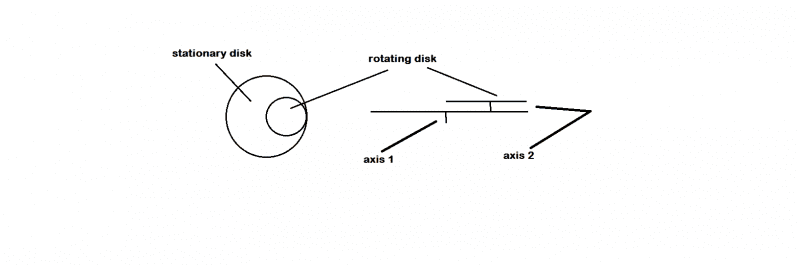# A doubt in angular momentum problem

## Homework Statement

if a disk is rotating on another stationary disk and someone standing on the stationary disk stops it what will the final angular velocity of both the disks be?
the catch is that both the disks are not co axial. assume ω angular velocity, M mass of big disk.m mass of small disk.R radius of larger disk,R/2 radius of small disk.I1=moment of inertia of big disk with respect to axis 1,I2=moment of inertia of small disk with respect to axis 2## Homework Equations

conservation of angular momentum

## The Attempt at a Solution

what is the angular momentum of the small disk with respect to the axis of the larger disk?

phinds
Gold Member
2021 Award
Your question as stated has a totally trivial answer. Since the question asks what is the final angular velocity of both disks after they are both stationary the answer is zero.

haruspex
Homework Helper
Gold Member
what is the angular momentum of the small disk with respect to the axis of the larger disk?
its definitely not I2ω since it would be angular momentum about axis 2.
Why do you think it cannot be the same?
You can represent a rotation about one axis as the sum of a linear motion and a rotation about another axis. Try doing that to express the smaller disk's rotation about axis 1.

so you mean total angular momentum of small disk about axis 1 is the sum of angular momentum of the disk about axis 2(here, assuming it does'nt rotate about axis 1) and the angular momentum of the small disk about axis1 (here, assuming it does'nt rotate about axis 2)?

haruspex
•